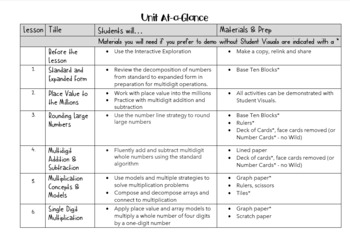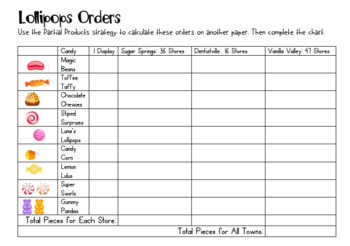# 4th Grade Place Value and Multidigit Operations--REMOTE edition

Rated 5 out of 5, based on 1 reviews
1 Rating;
4th - 5th
Subjects
Resource Type
Standards
Formats Included
•Google Drive™ folder
\$14.99
\$14.99
Report this resource to TPT#### What educators are saying

What a fantastic collection of activities to engage the students in meaningful practice and reinforcement while having fun!

### Description

This two+ week lesson plan pack comes with student printables, online activities, and digital versions of practice that will help your fourth graders tackle Number and Operations in Base Ten. It also includes ready-to-go Google Slideshows so you can demonstrate in a remote classroom environment, interactive games, as well as self-graded Google Form assignments!

This unit was designed for fourth-grade students and is fully aligned to the CCSS-M. It addresses these standards:

Generalize place value understanding for multi-digit whole numbers.

• Recognize that in a multi-digit whole number, a digit in one place represents ten times what it represents in the place to its right.
• Read and write multi-digit whole numbers using base-ten numerals, number names, and expanded form. Compare two multi-digit numbers based on meanings of the digits in each place.
• Use place value understanding to round multi-digit whole numbers to any place.

Use place value understanding and properties of operations to perform multi-digit arithmetic.

• Fluently add and subtract multi-digit whole numbers using the standard algorithm.
• Multiply a whole number of up to four digits by a one-digit whole number, and multiply two two-digit numbers, using strategies based on place value and the properties of operations. Illustrate and explain the calculation by using equations, rectangular arrays, and/or area models.
• Find whole-number quotients and remainders with up to four-digit dividends and one-digit divisors, using strategies based on place value, the properties of operations, and/or the relationship between multiplication and division. Illustrate and explain the calculation by using equations, rectangular arrays, and/or area models.

Total Pages
Included
Teaching Duration
2 Weeks
Report this resource to TPT
Reported resources will be reviewed by our team. Report this resource to let us know if this resource violates TPT’s content guidelines.

### Standards

to see state-specific standards (only available in the US).
Recognize that in a multi-digit whole number, a digit in one place represents ten times what it represents in the place to its right. For example, recognize that 700 ÷ 70 = 10 by applying concepts of place value and division.
Read and write multi-digit whole numbers using base-ten numerals, number names, and expanded form. Compare two multi-digit numbers based on meanings of the digits in each place, using >, =, and < symbols to record the results of comparisons.
Use place value understanding to round multi-digit whole numbers to any place.
Fluently add and subtract multi-digit whole numbers using the standard algorithm.
Multiply a whole number of up to four digits by a one-digit whole number, and multiply two two-digit numbers, using strategies based on place value and the properties of operations. Illustrate and explain the calculation by using equations, rectangular arrays, and/or area models.